## Year 5 addition of three 2-digit numbers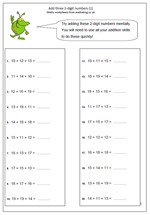Here is a practice page of adding three 2-digit numbers mentally. This is a really good test of how well children can use their skills and knowledge as there are several different approaches/techniques that can be used.

These skills  include:

1. Because addition can be done in any order it is often easier to start with the largest number.

2. Knowing pairs of teen numbers (e.g. knowing 17 + 17 is 34) will help with a question such as 17 + 15 + 17.

3. Looking for pairs that make a multiple of 10: (e.g. 17 + 13 = 30)

4. Adding the tens before the units and then counting on.

This page can be found in our Year 5 category but there are also a great collection in our Four Rules category.

## Bonfire Night Worksheets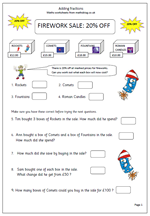It’s always nice to have something topical to use as part of the maths curriculum. Today I would like to point you to a page on percentages, linked to the theme of bonfire night, or fireworks, suitable for Year 6 children who are learning about how to work out percentages.

Four different kinds of fireworks are on sale, with 20% off. The questions involve working out how much the boxes cost and how much in the way of savings can be made.

Finding 20% is relatively straightforward if done in two parts:

first find 10% by dividing by 2

find 20% by doubling the answer.

You can find this page in my Year 6, Understanding Number section.

Thanks to urbrainy.com for letting me publish this page. There are more bonfire night pages on their site, which you can trial free of charge at urbrainy.com

Bonfire night percentages

## Understanding multiplication in year 1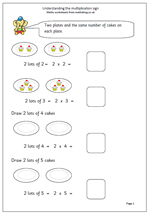At one time multiplication was not really introduced until year 2, but recent curriculum planning has included understanding multiplication into year 1, so here is a couple of pages to try to help with this.

At first multiplication can be seen as repeated addition so that:

2 added together 3 times is 2 + 2 + 2, or 3 lots of 2, or 3 times 2, or 3 x 2 (or 2 x 3).

The multiplication sign needs to be introduced, including using the words ‘times’, ‘multiply’ and ‘lots of’.

The second of the pages is quite a bit harder as there are no illustrations and various parts of the number sentences are missing. it would be a good idea to ‘talk through’ each number sentence, saying them out loud.

Understand the multiplication sign

## Counting maths activity for young children

This week we highlight a simple a little counting game suitable for young children. Ted just loves to watch football on the TV and when he is older he will be allowed to go and see his favourite team play. I wonder who his favourite team is? I bet it is the same as yours!

## Patterns and problems in Year 4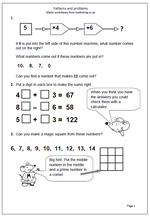There are many different ways of presenting number problems to children, other than just the traditional ‘sums’ style approach. This page shows three very different approaches to questions that many children will find quite hard.

The first is in the style of a function machine, i.e. a number is put into the machine, various operations are carried out and a number will come out at the end. For this function machine the operations are: x 4 and + 6. The hardest part of this is the last question where the output is given and the input has to be worked out and the machine can be reversed by subtracting 6 and dividing by 4 to get the answer, although many children will use trial and error/improvement methods.

The number sentences in the second question are quite straightforward apart from the fact that both tens and units digits are missing. it is easiest to take one part at a time e.g. find the missing unit and then the missing ten. Watch out for the subtraction!

By year 5 children should be used to magic squares and not find this too tricky, especially with the clue to help.

This page can be found in our year 4, Using and Applying Maths category.

Patterns and problems

## Short multiplication by 2, 5 and 10.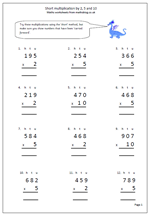When children are learning the standard methods of multiplication they need plenty of practice. With multiplication there are two distinct methods, the long and the short! Most schools tend to teach the long method first as it is clearer to show exactly what is happening, but it is also important for children to learn the short method, as we are looking to achieve fast accurate calculating.

This page keeps it simple by just multiplying by 2, 5 and 10; three tables that children should know ‘off by heart’.The questions have been laid out in the correct way so all that has to be done is:

a. Firstly to multiply by the units, remembering to carry any tens across to the tens column. These are usually placed under the answer.

b. Secondly multiply the tens remembering to carry any hundreds across to the hundreds column

c. Finally multiply the hundreds and write in the answer, including any thousands.

Short multiplication by 2, 5 and 10

## Worksheet on problems involving measurement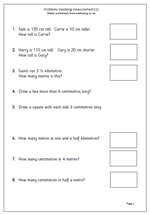By the end of year 3 children should be confident with using comparative terms such as taller, shorter, longest etc. They should know that:

1 kilometre = 1000 metres

1 metre = 100 centimetres

1 kilogram = 1000 grams

1 litre = 1000 millilitres

They should also begin to recognise simple fractions and convert between units, such as that 3 and a half kilometres is 3500 metres.

Rulers should be used to a fair degree of accuracy.

This worksheet covers some aspects of this and would be a good assessment or homework sheet.

Measurement problems

## Resource of the week: KS2 SAT style questions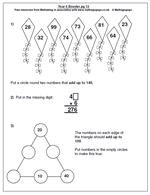It’s never too early to get to grips with some SAT style questions in Year 6 and we have an increasing number of these. There are two similar maths worksheets here which demonstrate a number of techniques that can be used to answer SAT questions.

The first question asks for two numbers which add up to 140 from the selection given. The easiest approach to this is firstly to look at the units and find two which add up to 10, such as 28 and 32. Then to look to see if the tens digits are suitable; in this case they are not.

Try again 99 and 91 – this time too big.

Try 74 and 66.

6 and 4 make 10. 70 and 60 make 130. Add 10 to 130 makes 140 – there is your answer!

For question 3 the order is important. Start with an edge of the triangle which already has two numbers in.

Booster maths worksheet 13

Booster maths worksheet 14

## 2 times table up to 12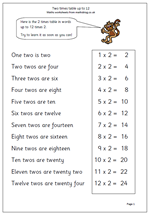Learning times tables off by heart is a fundamental part of primary maths, yet many children go on to High School without knowing them. This really inhibits progress with a whole range of other maths, including long and short  multiplication, long and short division, factors, multiples etc.

There are not that many facts to learn and once learned off by heart they are seldom forgotten.

Here are a couple of starter pages for the two times table. I have gone up to 12 x 2 because the new national Curriculum for maths seems to have this as one of its requirements next year, although many people would argue that learning tables up to 10 covers all necessary requirements.

Thanks to urbrainy.com for letting me use this resource from their wide range of worksheets.

2x table up to 12

## Written method for adding 4-digit numbers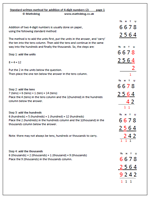Addition of two 4-digit numbers is usually done on paper,using the following standard method:

The method is to add the units first, put the units in the answer, and ‘carry’ the ten into the tens column. Then add the tens and continue in the same way into the hundreds and finally the thousands. So, looking at the addition of 6678 + 2564,

the steps are:

8 + 4 = 12

Put the 2 in the units below the question.
Then place the one ten below the answer in the tens column.
7 (tens) + 6 (tens) + 1 (ten) = 14 (tens)
Place the 4 (tens) in the tens column and the 1(hundred) in the hundreds column below the answer.